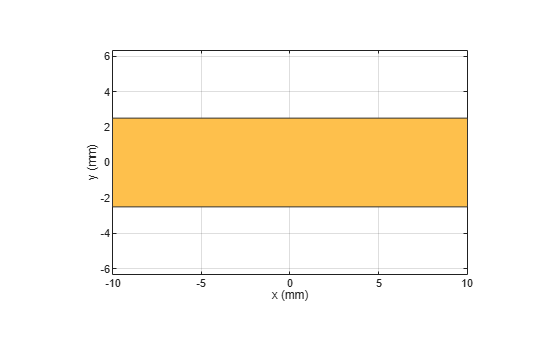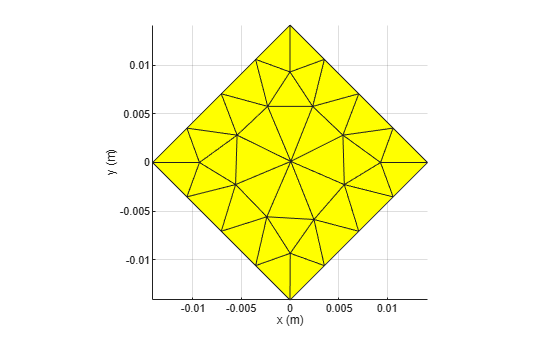# traceRectangular

Create rectangular trace

Since R2021b

## Description

Use the `traceRectangular` object to create a rectangular trace centered at the origin on the X-Y plane.

## Creation

### Syntax

``trace = traceRectangular``
``trace = traceRectangular(Name=Value)``

### Description

example

````trace = traceRectangular` creates a rectangular trace centered at the origin and on the X-Y plane.```

example

````trace = traceRectangular(Name=Value)` sets Properties using one or more name-value arguments. For example, `traceRectangular(Center=[1 1])` creates a rectangular trace centered at `[1 1]`. Properties not specified retain their default values.```

## Properties

expand all

Name of the rectangular trace, specified as a character vector or a string scalar.

Example: `trace = traceRectangular(Name="rectangletrace1")`

Data Types: `char` | `string`

Center of the rectangular trace in Cartesian coordinates, specified as a two-element vector.

Example: `trace = traceRectangular(Center=[1 1])`

Data Types: `double`

Length of the rectangle in meters, specified as a positive scalar.

Example: `trace = traceRectangular(Length=0.0500)`

Data Types: `double`

Width of the rectangle in meters, specified as a positive scalar.

Example: `trace = traceRectangular(Width=0.015)`

Data Types: `double`

## Object Functions

 `add` Boolean unite operation on two RF PCB shapes `and` Shape1 & Shape2 for RF PCB shapes `area` Calculate area of RF PCB shape in square meters `intersect` Boolean intersection operation on two RF PCB shapes `mesh` Change and view mesh properties of metal or dielectric in PCB component `minus` Shape1 - Shape2 for RF PCB shapes `plus` Shape1 + Shape2 for RF PCB shapes `rotate` Rotate RF PCB shape about defined axis `rotateX` Rotate RF PCB shape about x-axis `rotateY` Rotate RF PCB shape about y-axis and angle `rotateZ` Rotate RF PCB shape about z-axis `subtract` Boolean subtraction operation on two RF PCB shapes `scale` Change size of RF PCB shape by fixed amount `show` Display PCB component structure or PCB shape `translate` Move RF PCB shape to new location

## Examples

collapse all

Create a rectangular trace with default properties.

`trace = traceRectangular`
```trace = traceRectangular with properties: Name: 'mytraceRectangular' Center: [0 0] Length: 0.0200 Width: 0.0050 ```

View the trace.

`show(trace)`Create a 2 cm-by-2 cm rectangular trace.

`trace = traceRectangular(Length=0.02,Width=0.02);`

Rotate the trace by 45 degrees about the z-axis.

`trace = rotateZ(trace,45);`

Mesh the trace at a maximum edge length of 5 mm.

`mesh(trace,MaxEdgeLength=5e-3)`## Version History

Introduced in R2021b